## Op-Amps - An Introduction

Operational amplifiers, or op-amps for short, have been around for many years. There was a time when computing was performed by manipulating analogue voltages rather than the binary 0s and 1s of contempory computing. A general purpose amplifier "module" was designed for this application, and dozens of these operational amplifiers could be connected together, their individual functions depending on the exact configuration of the surrounding components.

An op-amp has 3 basic connection - two inputs and one output. Additionally, a power supply is required, but for the purposes of understanding, this can be ignored initially.

Why 2 inputs? An op-amp responds to the difference between the two inputs. If both the inputs are equal, there is no output. It doesn't matter if both the inputs are at say, 10 volts or 0 volts or -10 volts; if they are the same, there is no output.

One inputs is called the non-inverting input, or "+" for short. The other is called, not surprisingly, the inverting input, or "-". If the non-inverting input is higher than the inverting input, the output will be positive. Conversely, should the "+" input be lower than the "-" input, the output goes negative.

Having determined which direction the output moves in response to an input voltage difference, the next question is how far will it move? To determine this, we need to know the gain of the amplifier. A typical op-amp has a voltage gain of around a million - this means that 0.000,001V applied to the input will result in 1V on the output! Clearly, there is far more gain than we require, so we must "throw away" gain using a technique called negative feedback.

Negative feedback represents a rare occurance in engineering - a win-win situation. By sacrificing the voltage gain, every other parameter of the amplifier improves. Noise, distortion and frequency response, for example, all get better.

### What do op-amps look like?

An op-amp is just an amplifier circuit - as such it can take many physical forms. One could be constructed from discrete components - seperate resistors, capacitors and transistors. You could even build one using valves! But the most common implementation is seen in IC form - here are some examples:Most of the devices shown above actually include two op-amps in the package, convenient for helping to make practical realisations neat and compact. This is the schematic symbol for an op-amp: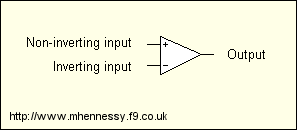### Op-amp "golden rules"

Taken straight from good text books (like The Art of Electronics by Horowitz and Hill). Remember these when analysing op-amp circuits, and understanding is easy:

1. The inputs are always at the same potential.
2. The inputs draw no current.

An important note regarding Rule 1 - this is only true when the op-amp is used in a correctly designed circuit that is being used within its limits. The op-amp obeys Rule 1 by moving its output terminal until it sees that the two inputs are the same. This can only happen when the external feedback network has been correctly configured.

### The non-inverting amplifier

This is a very common circuit in audio and other applications.How does this work? Let's assume that we have applied a signal to the input, and work out what the output should be. But first, consider that signal. It's conventional to assume that an audio signal can swing either side of a central point, which is considered to be ground or 0V. Sometimes this central point will be some other voltage, but don't worry about that for now (just remember the expression "DC offset").

So that we can analyse the operation, lets "freeze" the signal in time. Assume that it is 1V. Maybe it will be -1V at some other time, perhaps 0.5V after that. The theory holds true, whichever voltage you pick, so we'll pick 1V because, (a) it's not unrealistic, and (b) it makes the maths easy!

With 1V applied to the input of the circuit, the non-inverting input is clearly held at 1V above ground. Remember Rule 1 - the inputs are always at the same potential. Therefore, the non-inverting input must also be at 1V. So what is the output voltage?

We can work this out. RF and RI form a potential divider, and because of Rule 2, the maths here is really easy. As there is 1V across RI, and RI is 1K, then we know from Ohm's law that there must be 1mA flowing through RI. Because of Rule 2, there must also be 1mA in the feedback resistor RF - there is nowhere else for the current to go. Now, as RF is 3K, Ohm's laws tells us that there must be 3 volts across RF. So what is the output voltage? The sum of the voltages across RI and RF - in other words 4 volts.

So for an input of 1 volt, we get 4 volts out. Repeat the sums for 2 volts in, and 0.5 volts in. In each case, the ratio between Vin and Vout will be the same, and this ratio is called the gain of the amplifier.

Gain = 1 + (RF / RI)

The input impedance of this circuit is very high because the input draws no current. Although not shown above, it is normal to add a resistor between the non-inverting and ground - this will ensure the input is held at 0 volts with no signal (though often, some feature of the preceeding circuitry does this job). The input impedance is equal to this resistor - typical values range from a few Kohms, up 1M or so...

### Unity-gain buffer

This is a special case of the non-inverting amplifier shown above.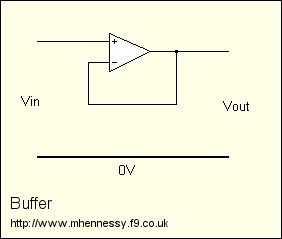This is simple to analyse - remembering Rule 1, we can see that the output will always be the same potential as the input because the inverting input is directly connected to the output. So we have a circuit with a gain of 1.

This might not seem particularly useful, but consider the input and output impedance of the circuit. We said before that the input impedance of a non-inverting amplifier is very high - this means that this amplifier doesn't place any "load" on the preceeding circuitry, which might be very useful in a number of circumstances. The output impedance of this circuit is very low because of the high level of negative feedback.

So this circuit presents a very easy load to the preceeding device, yet is able to drive a difficult load with ease. Although it has no voltage gain, it has current gain. An ideal buffer...

### The inverting amplifier

Another common op-amp circuit is the inverting amplifier. The schematic is shown here.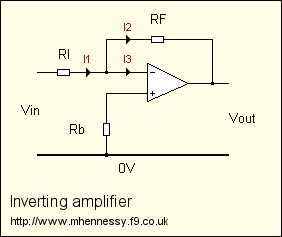To understand this circuit, we must remember both the "golden rules". Consider the non-inverting input - what is the voltage here?

To determine this we remember Rule 2 - there is no current flowing into the input - therefore Ohm's Law tells us that no voltage is dropped across Rb. Hence the non-inverting input is grounded. Some designs replace Rb with a short-circuit - given the right circumstances this can be perfectly acceptible.

Next, remember Rule 1 - the inputs are always the same. So when an input of say, 1 volt is applied to the input, the inverting input is at 0 volts. Yet we can get a signal through this amplifier - how is this possible?

We need to consider the currents flowing in the resistors. Suppose RI is 1K, and 1 volt is applied to the input. Therefore, 1mA flows through RI - this current is labelled I1. At the inverting input we have a junction, and Kirchoff states that current entering a junction equals current leaving a junction. But we know from Rule 2 that no current flows into the inputs, therefore I3 must be zero. So I2 equals I1 - the current in both resistors is the same.

Assuming again that RF is 3K, then Ohm's Law tells us that there will be 3 volts across it. Due to the direction of the flowing current, the output voltage is actually negative.

Increasing the input will cause the potential at the inverting input to rise. The op-amp will sense a difference between its two inputs, and the output will respond by moving more negative. It will continue to move negative until the inverting input reaches 0 volts again. Similarly, decreasing the input voltage will make the op-amp output rise because it sees its inverting input falling. Again, the output will stop rising when equilibrium is restored. You can think of the action as a see-saw: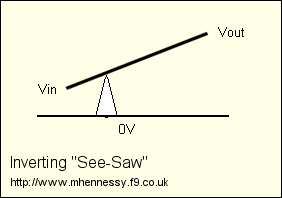The pivot point is placed such that the right side is three times longer than the left - this represents our choice of 1K and 3K input and feedback resistors.

Gain = - (RF / RI)

The input impedance is equal to RI - this is because the inverting input is held at 0 volts by negative feedback. For this reason, the point is called a "virtual earth".

### Other op-amp circuits

The following circuits are encountered in audio applications, but are slightly less common than the circuits covered so far. Therefore the explanations will be brief, but should you be curious any good text book will help you out.

#### The differential amplifier

This circuit has two inputs, and responds to the difference between them. Sounds familiar?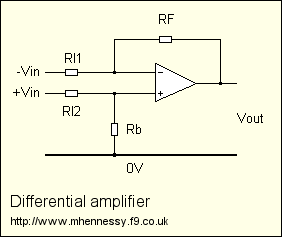This circuit is commonly used in audio applications to convert a balanced signal into a convential, single-ended signal. Nearly all domestic domestic audio systems use single-ended signals, although there are exceptions. If you want to build this circuit, perhaps because you want to interface with professional audio equipment, I recommend starting out making all the resistors 10K or thereabouts. I will leave it up to you to determine the impedance or each input, but suffice to say that one of the results is surprising.

#### The summing amplifier

This amplifier makes use of the virtual earth present in an inverting amplifier.The signal voltages are converted to currents by the input resistors, and these currents are summed at the virtual earth. The ratio of these resistors determines how the signals are mixed. This simple circuit forms the basis of mixing desks. You can use this circuit to add together the left and right signals of a stereo source to produce a mono mix.

### Power supplies

We've ignored these up to this point, but clearly any amplifier needs a source of power. Op-amp circuits use a split supply - two supply rails that are symetrical around 0 volts.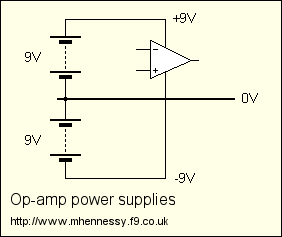This diagram demonstrates how to use two 9 volt batteries to make a split supply. The total supply voltage to the op-amp is 18 volts, but as voltages are generally measures with respect to earth or 0 volts, it's normal to write +9V and -9V as shown.

Of course, these batteries can be replaced with a suitable mains-powered supply, and these are the subject of another article.

Split supplies are the norm for op-amp circuits because signals generally swing either side of ground - this arrangement passes the signals easily and logically. But it is possible to design op-amp circuits that run from a single supply rail. This is convenient for portable operation, saving the cost of expensive batteries.

### Op-amp specifications

For the purposes of understanding, we assumed that the op-amps used so far have been "perfect". In practice, however, there are inherent restrictions and compromises on the exact performance, and you need to have an awareness of these when designing your own circuits.

So what are the ideal specifications for a "perfect" op-amp?

1. Input impedance is infinity.
2. Output impedance is zero.
3. Voltage gain is infinity.
4. Common-mode voltage gain is zero.
5. Output voltage is zero when both inputs are equal.
6. Output can change instantaneously.
7. Output will not be affected by the supply rails.

So what exactly do these mean, and how do practical op-amps differ from this?

#### 1. Input impedance

Because of the high impedance of the op-amps' input stage, combined with "bootstrapping" effects caused by negative feedback, the input impedance of an op-amp is infinite for all practical purposes. A more important consideration is the effects of input currents, which is a DC-precision issue:

Practical op-amps draw a small DC current from the source. For bipolar devices (op-amps that use conventional BJT transistors for the input stage) such as the NE5534 or LM388, this current will be in the order of 1 to 2uA (micro-amps). A FET-input op-amp like the OPA134 or OPA604 will have a much smaller input current - measured in pica-amps - although this varies with temperature.

This "bias current" is important to consider when choosing the feedback and other resistors that connect to the inputs of op-amps, because unwanted DC-offsets can result. As a general rule, try to ensure that both inputs "see" the same resistance. Consider these examples: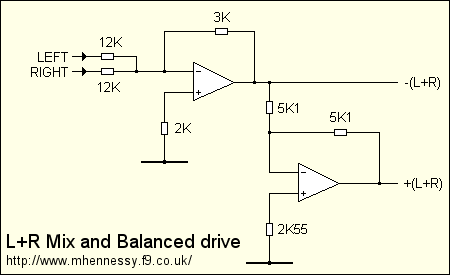The inverting input of the first op-amp "sees" the two 12K input resistors, and the 3K feedback resistor. The inputs come from a low-impedance source, so we can assume that the are effectively connected to ground (via a perfect voltage source). The output of the op-amp also approximates a perfect voltage source, so again we can regard the feedback resistor as being connected to ground. Therefore, all three resistors appear to be in parallel, and the combined parallel resistance of two 12K resistors and the 3K resistor works out to be exactly 2K. Hence, the non-inverting input is connected to ground via a 2K resistor.

Assume that an op-amp was used that had 1uA bias currents. The 1uA flowing in the 2K resistances will develop a 2mV potential difference across them. Suppose that we'd connected the non-inverting input directly to ground - this means that a 2mV potential difference will exist between the inputs, and remember that the op-amp responds to the difference between the inputs. Result? DC offset.

The same princible was applied in the following op-amp, which is a simple inverter with a gain of -1. The two 5K1 resistors set this gain, and this time the non-inverting input requires a 2.55K resistance to ground.

Input current is quoted on datasheets, and is rarely a serious issue for audio applications, provided you take the measures demonstrated above. Sometimes this isn't practical, so the issue can be avoided by choosing a FET-input op-amp with much lower input currents. However, this can become significant with chip-amp power amplifiers, where the gain is typically higher than in pre-amp circuits, and there is less flexibility with the circuit configuration.

A related issue is input offset current, which is the name given to the difference between bias currents for each input. This is as a result of manufacturing variations, and is hard to predict. Typically, the offset might be around 10-50% of the bias current - check the datasheets. This won't cause you a serious problem for one-off projects, but it's something to consider if you're planning to go into production!

#### 2. Output impedance

This refers to the internal resistance of the output stage of the op-amp. Devices such as batteries, power supplies, signal sources and amplifiers are frequently modelled as a perfect voltage source with some internal resistance, Ro (or Zo). When a load is connected to the output, the output voltage will fall because the load forms a potential divider.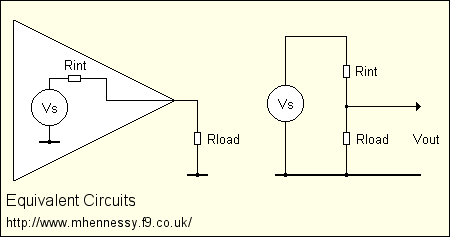Typical values of Rint range from less than an ohm to several K, depending on the device. Negative feedback will reduce the effective internal resistance to less than an ohm in a typical audio circuit.

Quite apart from Rint, the drive capability of the op-amp is important. This refers to the amount of current that an op-amp can source or sink, and staying within this limit is vitally important to ensuring the long-term reliability of your design. Typically values range from around 10mA for a TL071 or 40mA for an NE5534, rising to 10 amps plus for an LM4780.

#### 3. Voltage gain

The voltage gain of an op-amp at DC is around 1,000,000. For typically internally-compensated op-amps, this gain falls as frequency rises at a rate of 6dB's per octave, reaching unity at a frequency known as fT. This is known as the GBP (gain-bandwidth product). For example, the LM833 has a GBP of 15MHz - suppose that you built a circuit with a gain of ten. The bandwidth of this amplifier will be 1.5MHz. Increase the gain to 100 and the bandwidth will be 150KHz.

This is rarely a problem with audio circuits, unless you're building microphone preamps or MC RIAA phono stages. Good audio op-amps have a GBP of around 10MHz or more. Beware of selecting excessively fast op-amps - unless you're very careful you will run into all sorts of problems!

#### 4. Common-mode voltage gain

We started this page saying that op-amps respond to the difference between the two inputs. Therefore, by implication, the op-amp must ignore voltages that are common to both inputs. This is called as CMRR, or common-mode rejection ratio.

This parameter is less important for most audio applications, and is typically dictated by the surrounding circuit conditions than the actual op-amp itself. Typical values for CMRR are around 90-130dB. Note that this falls with fequency.

#### 5. Zero offset voltage

When both inputs are tied together, the output of the op-amp should be zero, but owing to tolerances in manufacturing, this is rarely the case. Offset voltage, Vos, is the voltage that is required to achieve an output of 0V, and it typically ranges from 0.1 to 15 mV.

This is yet another factor that contributes to DC offsets in a system. It varies with temperature, time and device sample. As with offset currents, it rarely causes insurmountable problems in one-off projects, but needs careful engineering for volume production.

#### 6. Output slew rate

An ideal op-amp can alter its output instantaneously but in practice a limitation called slew rate dictates how quickly an op-amp can change output voltage. This is a rate-of-change measured in volts per microsecond (V/us). Typical values range from 1 to 20V/us for audio op-amps, much higher numbers are available for high-speed video op-amps.

Slew rate is rarely an issue for line-level applications, but can become important for power amplification. As for typical minimum values, opinion varies wildly. You can calculate that a 100 watt amplifier would need a minimum slew rate of 5V/us to reproduce a 20KHz sinewave, so 10V/us amplifier would have a reasonable safety margin. All the available audio power amplifier ICs comfortably exceed these recomendations - for example the LM4780 offers 19V/us.

#### 7. Power supply immunity

Disturbances on the power supply rails should not appear on the output. This is called power supply rejection ratio (PSRR), and is quoted in dB's. Typical numbers range from 60 to 120dB, but note that this falls with frequency at typically 6dB per octave.

This is important for power amplifiers when they are powered by a simple unregulated supply which will contain high levels of 100Hz ripple. In theory, 2 volts of ripple attenuated by 80dB will appear on the output as 0.2mV. In practice there are other factors, so it is important to ensure that op-amp power supplies are as clean and noise-free as possible.